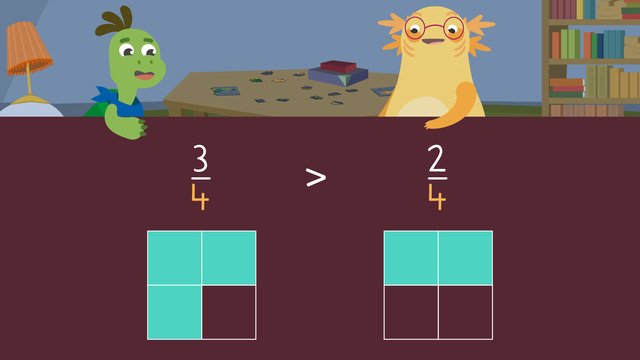# Fractions with the Same Denominator

Content Fractions with the Same DenominatorRating

Ø 5.0 / 2 ratings
The authorsTeam Digital
Fractions with the Same Denominator
CCSS.MATH.CONTENT.3.NF.A.3.D

## Comparing Fractions with Like Denominators

Before we compare fractions with the same denominators, we must think about what a denominator means. Denominators show how many equal parts a whole is divided into. Fractions with the same denominators are called like fractions as long as the whole is identical.The fraction with the greater numerator will always be larger when comparing like fractions from the same whole. This is because each part is the same size, and the greater numerator is telling us we have more of the equal parts. Fraction models can help us see if like fractions are greater than, less than, or equal to one another. How do you compare fractions with like denominators? Read on below to learn how!

## Comparing Fractions with the Same Denominator – Steps

Let's practice comparing the like fractions three fourths and two fourths. They are like fractions because both have the same denominator and come from the same whole. First, draw and shade a fraction model to represent three fourths.Then, draw and shade another identical fraction model to represent two fourths.Finally, compare the shaded values of both models.Three fourths has more parts shaded than two fourths. So three fourths is greater than two fourths. Remember, the like fraction with the greater numerator is larger.That is how to compare fractions with the same denominator but different numerators.

## Comparing Fractions with the Same Denominator – Summary

These are the steps for comparing fractions with the same denominator:

Step # What to do
1 Draw and shade a fraction model to represent
the first fraction.
2 Draw and shade another identical fraction
model to represent the second fraction.
3 Compare the shaded values of both models.
4 The fraction with more shaded parts is
greater!

Have you practiced yet? On this website, you can also compare fractions with the same denominator with worksheets and exercises.

### TranscriptFractions with the Same Denominator

1 comment
1 comment
1.I love this program it is so fun

From Alaina, 8 months ago

## Fractions with the Same Denominator exercise

Would you like to apply the knowledge you’ve learned? You can review and practice it with the tasks for the video Fractions with the Same Denominator.
• ### When comparing like fractions with identical wholes, the fraction with the greater numerator will always be _______.

Hints

Remember like fractions have the same denominator.

Which fraction has more equal parts shaded in here?

Remember, each part is the same size and the greater numerator is telling us we have more of the equal parts.

Solution

When comparing like fractions with identical wholes, the fraction with the greater numerator will always be larger

You can see this in the image above. Each part is the same size and the greater numerator is telling us we have more of the equal parts.

• ### Which expressions are true?

Hints

Remember when comparing like fractions (with the same denominator) that also have the same whole, the larger numerator will always be the larger fraction.

< means less than and > means greater than.

When looking at the fraction models, which fraction has more equal parts shaded in? That will be the larger fraction.

Solution

Shown are the correct expressions. Remember, when comparing like fractions with the same whole, the larger numerator will also be the larger fraction.

The first expression is incorrect because $\frac{1}{3}$ is < $\frac{2}{3}$

The second expression is correct because $\frac{3}{6}$ is < $\frac{4}{6}$

The third expression is incorrect because $\frac{7}{8}$ is > $\frac{6}{8}$

The fourth expression is correct because $\frac{5}{6}$ is > $\frac{4}{6}$

• ### Comparing fractions.

Hints

Remember when comparing like fractions (with the same denominator) that also have the same whole, the larger numerator will always be the larger fraction.

< means less than and > means greater than.

When looking at the fraction models, which fraction has more equal parts shaded in? That will be the larger fraction.

Solution

Remember, when comparing like fractions with the same whole, the larger numerator will also be the larger fraction.

$\frac{3}{3}$ > $\frac{2}{3}$

$\frac{1}{6}$ < $\frac{2}{6}$

$\frac{2}{8}$ < $\frac{3}{8}$

$\frac{6}{8}$ > $\frac{5}{8}$

• ### Greater than or less than?

Hints

Remember when comparing like fractions that also have the same whole, the larger numerator will always be the larger fraction.

< means less than and > means greater than.

Solution

$\frac{5}{6}$ < $\frac{6}{6}$

$\frac{4}{6}$ < $\frac{5}{6}$

$\frac{2}{8}$ > $\frac{1}{8}$

$\frac{4}{8}$ > $\frac{3}{8}$

• ### What are like fractions?

Hints

Like fractions need to have the same whole.

When comparing like fractions we compare the numerators.

Here are 2 like fractions.

Solution

Like fractions are fractions that have the same whole and the same denominator.

Shown is an example of like fractions.

• ### Who ate more pizza?

Hints

< means less than and > means greater than.

The second set of fractions don't have the same size whole, so you need to look at the size of the pieces.

Make sure you're looking at how many pieces are shaded in.

Solution

For the first set, they got the same size pizza so, $\frac{2}{5}$ < $\frac{3}{5}$

For the second set, they didn't get the same size pizza so, $\frac{1}{4}$ > $\frac{2}{4}$ because the size of the pieces were larger.

For the third set, they got the same size pizza so, $\frac{4}{8}$ = $\frac{4}{8}$